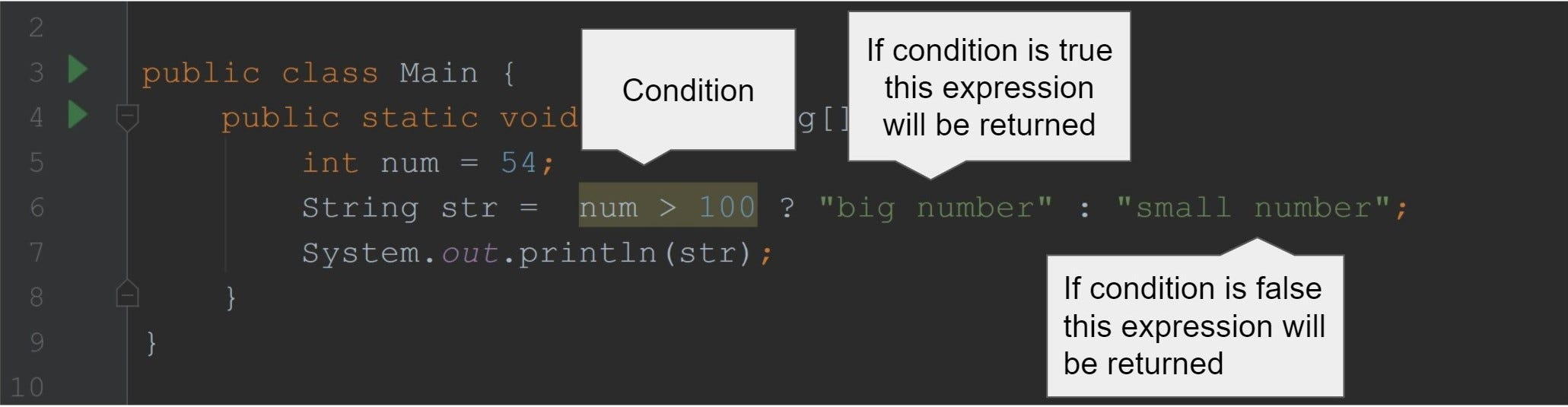# Ternary operator in Javaoutput is small number

The ternary operator is a simple conditional branching statement. It returns a value based on condition.

`int num = 54;String str = num > 100 ? "big number" : "small number";`

`num > 100 ?` is our condition.

`"big number"` the first value(before :) will be returned if a condition is true.

`"small number"` the second value(after :) will be returned if a condition is false. In our case, this value is returned because the condition is false.

Turnery operator can be used with any other data types as well.

`public class Main {    public static void main(String[] args) {        boolean b = false;        int num = b ? 7 : 9;        System.out.println(num); // 9    }}public class Main {    public static void main(String[] args) {        double score = 75.0;        char grade = score >= 50&& score <= 100 ? 'A' : 'F';        System.out.println(grade); // A    }}public class Main {    public static void main(String[] args) {        int num = 1 > 2 ? 7 : 9 > 3 ? 0 : 3;        System.out.println(num); // 0    }}`

## More from Beknazar

Software Developer, Java Instructor https://www.techleadacademy.io/